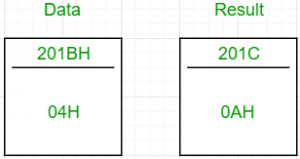GFG App
Open AppBrowser
Continue

# 8085 program to find the sum of first n natural numbers

Problem – Write an assembly language program for calculating the sum of first n natural numbers using 8085 microprocessor. Example –

Input : 04H
Output : 0AH
as 01+02+03+04 = 10 in decimal => 0AHThe formula for calculating the sum of first n natural numbers is. Algorithm –

1. With n as the input, increment it to obtain n+1.
2. Multiply n with n+1.
3. Divide the product obtained by 2.

In 8085 microprocessor, no direct instruction exists to multiply two numbers, so multiplication is done by repeated addition as 4×5 is equivalent to 4+4+4+4+4 (i.e., 5 times). Input: 04H Add 04H 5 times Product: 14H(2010) Similarly, in 8085 microprocessor, no direct instruction exists to divide two numbers, so division is done by repeated subtraction. Input: 14H Keep subtracting 2 from the input till it reduces to 0. Since subtraction has to be performed 1010 times before 14H becomes 0, the quotient is 1010 => 0AH. Steps –

1. Load the data from the memory location (201BH, arbitrary choice) into the accumulator
2. Move this data into B
3. Increment the value in the accumulator by one and move it to the register C
4. Initialise the accumulator with 0
5. Multiplication: Keep adding B to accumulator. The number of times B has to be added is equal to the value of C
6. Initialise B with 00H. B will store the quotient of the division
7. Initialise C with 02H. This is the divisor for the division
8. Division: Keep subtracting C from A till A becomes 0. For each subtraction, increment B by one
9. The final answer is in B. Move it to A. Then store the value of A in 201CH (arbitrary choice again)

Store the value of n in 201BH. The sum can be found at 201CH.

### Advantages of finding the sum:

• It is a fundamental arithmetic operation that is widely used in mathematics, physics, and engineering.

• It can be easily calculated using a formula or a loop in a programming language, making it a versatile tool for solving problems.

• It can provide insights into the properties and characteristics of a sequence of numbers, such as their average or variance.

### Disadvantages of finding the sum:

• It may not be practical for very large values of n, as the result can quickly become very large and difficult to represent or manipulate.

• It may not be useful in certain contexts where addition is not relevant or meaningful, such as in analyzing the frequencies of letters in a text.

• It may require specialized knowledge or software to handle large values of n, leading to computational complexity and potential errors.
My Personal Notes arrow_drop_up